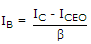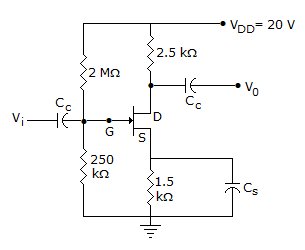# Electronics and Communication Engineering - Analog Electronics

36.

The units of transistor h parameters h11 and h22 are the same.

 A. True B. False

Explanation:

The units of h11 are ohms and units of h22 siemens.

37.

Positive feedback is mainly used in

 A. oscillators B. thyristors C. voltage regulators D. both (a) and (b)

Explanation:

In oscillators, the oscillations are generated due to positive feedback. In thyristors anode current increases due to positive feedback.

38.

For a BJT if β = 50, ICEO = 3 μA and IC = 1.2 mA then IB

 A. 24 μA B. 23 μA C. 20 μA D. 10 μA

Explanation:.

39.

In figure, ID = 4 mA. Then VD =A. 20 V B. 10 V C. 30 V D. 1 V

Explanation:

VD = 20 - 4 x 10-3 x 2.5 x 103 = 10 V.

40.

The main application of enhancement mode MOSFET is in

 A. amplifier circuits B. oscillator circuits C. switching circuits D. all of the above